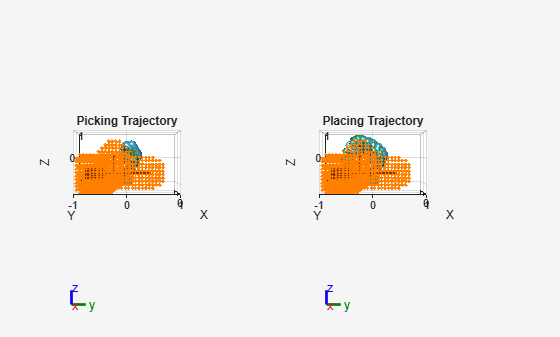# Pick-And-Place Workflow Using CHOMP for Manipulators

This example shows how to use CHOMP for manipulators to pick and place objects while simultaneously avoiding obstacles using the `manipulatorCHOMP` object. This example also shows some of the key differences between the RRT-based and CHOMP-based motion planning approaches to solving a pick-and-place task.

### Introduction

Covariant Hamiltonian Optimization for Motion Planning (CHOMP) is a trajectory-optimization-based motion planner that optimizes trajectories for smoothness and collision-avoidance. It does this by minimizing a cost function, comprised of a smoothness cost and a collision cost. Notable differences between the CHOMP and RRT algorithms are:

• CHOMP outputs a trajectory comprised of waypoint samples with associated timestamps, while RRT is a geometric planner that outputs waypoints without any associated times.

• CHOMP models collision avoidance as a cost, so minimizing the cost of collision is one of the objectives of the algorithm, whereas RRT does not include an edge (interpolations between two waypoints) in the resultant path if the edge is colliding. This means that you can obtain colliding trajectories from CHOMP depending on conditions and solver settings.

• While planning a path using RRT, the planner queries only whether a waypoint is colliding or not. When planning a path using CHOMP, the algorithm requires the gradient of the collision cost of a waypoint. The gradient of the collision cost is the change in collision cost when there is a minor change in the waypoint. This means that you must model the environment as spheres for CHOMP, and as convex collision geometries for RRT.

This example shows you how to load a manipulator robot and a pick-and-place environment, model convex collision geometries as spherical obstacles for CHOMP, tune `manipulatorCHOMP`, and visualize the resulting pick-and-place trajectories.

Use the `exampleHelperLoadPickAndPlaceCHOMP` helper function to load a Franka Emika Panda manipulator robot model and slightly modify the gripper. The helper function also loads collision objects to act as the environment and returns a starting configuration. The starting conditions and environment are similar to the setup found in the Pick and Place Using RRT for Manipulators example.

`[franka,startConfig,env] = exampleHelperLoadPickAndPlaceCHOMP;`

### Create Spherical Approximation of Environment

While the environment `env` is ready for use with an RRT motion planner, because CHOMP requires the robot, environment, and obstacles to be represented as spheres, you must first approximate the collision geometries in `env` as spheres. This figure shows the difference in setup between using RRT and using CHOMP. Notice the overlaid spherical approximations, which CHOMP uses for collision costs, over the collision geometries of the robot.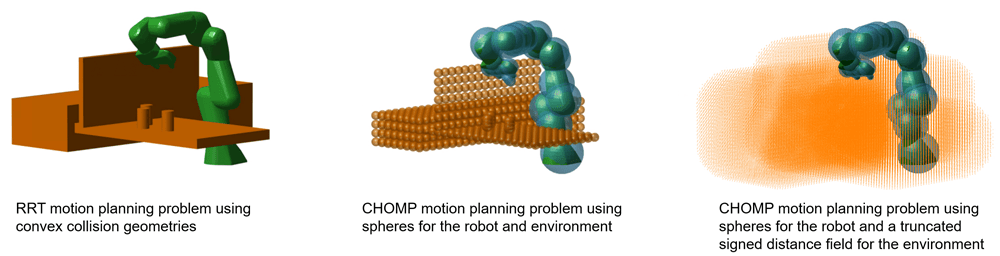In this example, `env` is a cell array of collision geometries, represented as `collisionBox` and `collisionCylinder` objects. To ensure the optimization routine accounts for the collision geometries, create an array, `sphobstacles`, in which to store the approximated environment.

`sphobstacles = [];`

The objective of this example is to pick the third object in the `env` cell array, a soda can, and place it over the barricade on the other side of the platform. This process physically moves the soda can from one place to another. From the perspective of a motion planning problem, you must remove the spheres that represent the soda can from the spherical obstacles and attach them to the gripper such that they become a part of the spheres on the rigid body tree. Create an array to track the spheres associated with the object to be picked via `spheresOfObjectToPick`, and another for tracking the indices of these spheres in the `sphobstacles` array, `spheresOfObjectToPickIdx`.

```indexOfCollisionObjectInEnvironmentToPick = 3; spheresOfObjectToPickIdx = []; spheresOfObjectToPick = [];```

The environment cell array consists of collision boxes that represent the platforms and barricades, and collision cylinders that represent the soda cans to be picked and placed. The `exampleHelperApproximateCollisionCylinderSpheres` and `exampleHelperApproximateCollisionBoxSpheres `helper functions approximate an array of spheres for the box and sphere collision geometry types, respectively.

• To approximate the collision cylinders using spheres, for each collision cylinder in the environment, fit a collision capsule using the `fitCollisionCapsule` object function, and then generate spheres on the capsule using `genspheres` at a ratio of `[0 0.5 1]`. This ratio generates a sphere at the beginning, middle, and end of the capsule. To more closely approximate the capsule, you can add more uniformly sampled ratio points.

• To approximate spheres on a collision box, use the `meshgrid` function. Define the grid over the length, height, and width of the box, and place a sphere at each grid point. Assume 20 spheres per unit length of the box. Increasing this density creates more spheres, which can more accurately model a box, but results in higher computation times when optimizing a trajectory.

```sphPerUnit = 20; % Spheres per unit length of box for i = 1:length(env) if(isa(env{i},"collisionCylinder")) ratio = [0 0.5 1]; sph = exampleHelperApproximateCollisionCylinderSpheres(env{i},ratio); else rho = 1/sphPerUnit; sph = exampleHelperApproximateCollisionBoxSpheres(env{i},rho); end if(i == indexOfCollisionObjectInEnvironmentToPick) spheresOfObjectToPickIdx = size(sphobstacles,2)+(1:size(sph,2)); spheresOfObjectToPick = sph; end sphobstacles = [sphobstacles sph]; end```

### Create Planner Instance

Initialize both the picking and placing configurations. The picking configuration places the end effector of the robot over the desired soda can, and the placing configuration places the end effector on the other side of the barricade.

```pickConfig = [0.2367 -0.0538 0.0536 -2.2101 0.0019 2.1565 -0.9682]; placeConfig = [-0.6564 0.2885 -0.3187 -1.5941 0.1103 1.8678 -0.2344];```

Create a `manipulatorCHOMP` object from the robot rigid body tree, and set the `SphericalObstacles` property to the spherical approximation of the environment.

```chomp = manipulatorCHOMP(franka); chomp.SphericalObstacles = sphobstacles```
```chomp = manipulatorCHOMP with properties: RigidBodyTree: [1x1 rigidBodyTree] RigidBodyTreeSpheres: [12x1 table] SmoothnessOptions: [1x1 chompSmoothnessOptions] SolverOptions: [1x1 chompSolverOptions] CollisionOptions: [1x1 chompCollisionOptions] SphericalObstacles: [4x1133 double] ```

For a collision-free trajectory, set a higher weight on the cost of collision as compared to smoothness. Increase the learning rate for higher convergence. Refer to the Tune Optimizer section to see the relationship between the various cost and solver options.

```chomp.CollisionOptions.CollisionCostWeight = 10; chomp.SmoothnessOptions.SmoothnessCostWeight = 1e-3; chomp.SolverOptions.LearningRate = 5;```

Visualize the starting, picking, and placing joint configurations of the robot for the CHOMP instance.

```show(chomp,[startConfig; pickConfig; placeConfig]); title("Starting, Picking, and Placing Configurations") xlim([-1 1]) ylim([-1 1]) zlim([-0.2 1])```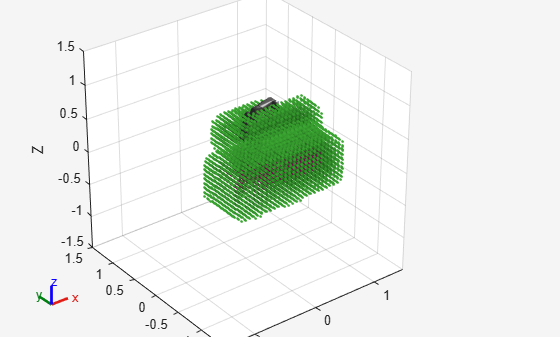#### Compare Motion Planning Problems

Visualize the differences in the collision models for the environment and the robot between the CHOMP and RRT solvers.

```subplot(1,2,1) show(franka,startConfig,Collisions="on",Visuals="off"); hold on for i = 1:length(env) show(env{i}); end hold off xlim([-1 1]) ylim([-1 1]) zlim([-0.2 1]) title("RRT Motion Planning Problem") subplot(1,2,2) show(chomp,startConfig); xlim([-1 1]) ylim([-1 1]) zlim([-0.2 1]) title("CHOMP Motion Planning Problem")```### Plan Picking Trajectory

Plan for an optimal collision-free picking trajectory. Assume a minimum-jerk trajectory between the starting and picking configurations. Additionally, assume the trajectory is 2 seconds long and is discretized in 0.1 second time steps.

```[optimpickconfig,timestamppick,solpickinfo] = optimize(chomp,[startConfig; pickConfig], ... % Starting and ending robot joint configurations [0 2], ... % Two waypoint times, first at 0s and last at 2s 0.10, ... % 0.1s time step InitialTrajectoryFitType="minjerkpolytraj");```

Display the `IsCollisionFree` and `InitialCollisionCost` fields of the `solpickinfo` structure, as well as the final collision cost of the `CollisionCost` field. Note that the `IsCollisionFree` field is `true`, meaning the trajectory is collision free, and the final collision cost of `CollisionCost` is lower than the initial collision cost. CHOMP is an optimization-based motion planner, so CHOMP treats the collisions during a trajectory as a cost and not a constraint. For more information on how CHOMP can output trajectories that result in collisions if you set weights inappropriately, see the Tune Optimizer section.

`solpickinfo.IsCollisionFree`
```ans = logical 1 ```
`solpickinfo.InitialCollisionCost`
```ans = 0.0016 ```
`solpickinfo.CollisionCost(solpickinfo.Iterations)`
```ans = 5.7837e-06 ```

Show the optimized configuration trajectory between the starting configuration and the picking configuration.

```figure show(chomp,optimpickconfig); title("Optimized Picking Trajectory") xlim([-1 1]) ylim([-1 1]) zlim([-0.2 1])```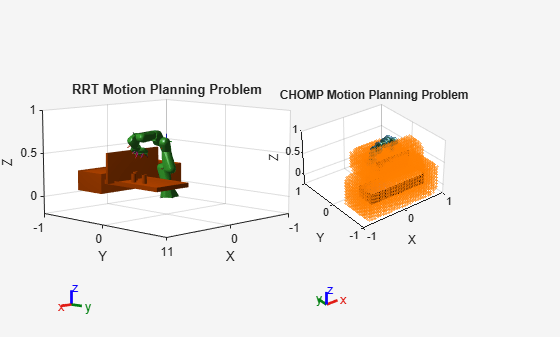### Plan Placing Trajectory

Remove the can as an obstacle in the CHOMP environment and get the transformation from the base of the robot to the hand of the robot. Then transform the spheres of the can so that they are in the end-effector frame, and add the spheres of the can to the cell array of spheres of the robot hand.

```chomp.SphericalObstacles(:,spheresOfObjectToPickIdx) = []; Tee = getTransform(franka,pickConfig,"panda_hand"); spheresInEeFrame = transform(inv(se3(Tee)),spheresOfObjectToPick(2:end,:)')'; pandahandspheres = chomp.RigidBodyTreeSpheres("panda_hand","spheres").spheres{1}; chomp.RigidBodyTreeSpheres("panda_hand","spheres").spheres{1} = [pandahandspheres,[spheresOfObjectToPick(1,:);spheresInEeFrame]];```

Plan the placing trajectory.

```[optimplaceconfig,timestampplace,solplaceinfo] = optimize(chomp,[pickConfig; placeConfig], ... [0 2], ... 0.10, ... InitialTrajectoryFitType="minjerkpolytraj");```

Note that the `InitialCollisionCost` is higher than the final collision cost, which is zero, and that the trajectory is collision free.

`solplaceinfo.InitialCollisionCost`
```ans = 0.8179 ```
`solplaceinfo.CollisionCost(solplaceinfo.Iterations)`
```ans = 0 ```
`solplaceinfo.IsCollisionFree`
```ans = logical 1 ```

Show the optimized configuration trajectory between the picking configuration and the placing configuration.

```figure show(chomp,optimplaceconfig); title("Optimized Placing Trajectory") xlim([-1 1]) ylim([-1 1]) zlim([-0.2 1])```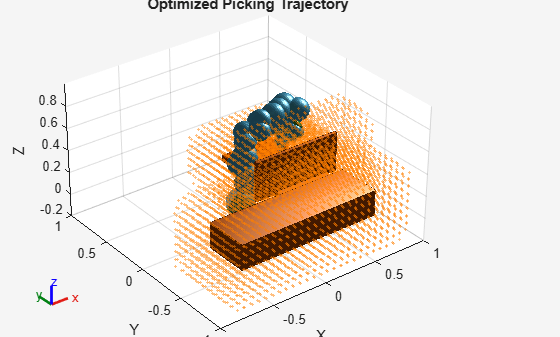### Tune Optimizer

CHOMP tries to find a balance between collision avoidance and smoothness a trajectory. The `chompCollisionOptions`, `chompSmoothnessOptions`, and `chompSolverOptions` objects help you specify how the solver should weigh these costs. These are the three parameters that you can tune to obtain an optimal trajectory:

• `CollisionCostWeight` — A property of the `chompCollisionOptions` object, this value specifies how much weight the optimizer should place on the collision avoidance cost of the trajectory. This weight affects the rate of change of cost of collision of the trajectory per iteration. A higher value indicates a higher change per iteration in the collision cost.

• `SmoothnessCostWeight` — A property of the `chompSmoothnessOptions` object, this value specifies how much weight the optimizer should place on smoothness of the trajectory. This weight affects the rate of change of the smoothness cost of the trajectory per iteration. A higher value indicates higher change per iteration in the smoothness cost.

• `LearningRate` — A property of the `chompSolverOptions` object, this value specifies how the overall objective function, such as the sum of the weighted smoothness and collision costs, changes per iteration. A higher value indicates the trajectory updates faster per iteration.

#### Decrease Collision Cost Weight

Decrease the value of the `CollisionCostWeight` property and plan the placing trajectory again.

```chomp.CollisionOptions.CollisionCostWeight = 1.5; [placetraj,~,lowcollcostinfo] = optimize(chomp,[pickConfig; placeConfig], ... [0 2], ... 0.10, ... InitialTrajectoryFitType="minjerkpolytraj");```

Plot the results of the planner with a collision cost weight of `10` and `1.5` to see the effect. Note that setting the collision cost weight to a lower value results in a higher collision cost when the optimizer completes its trajectory planning.

```figure plot([solplaceinfo.InitialCollisionCost,solplaceinfo.CollisionCost]) hold on plot([lowcollcostinfo.InitialCollisionCost,lowcollcostinfo.CollisionCost]) title("Effect of Decreasing Collision Cost Weight") legend({"CollisionCostWeight=10","CollisionCostWeight=1.5"}) xlabel("Iterations") ylabel("Collision Cost") hold off```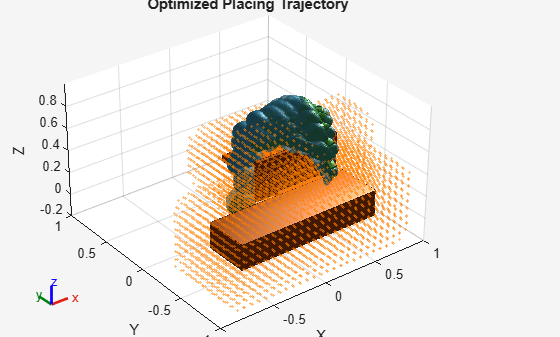Display the `IsCollisionFree` field of the solution info, and show the trajectory with this lower collision cost weight. Notice that the trajectory is no longer collision free after decreasing the collision cost weight to `1.5`.

`disp(lowcollcostinfo.IsCollisionFree)`
``` 0 ```
```show(chomp,placetraj); xlim([-1 1]) ylim([-1 1]) zlim([-0.2 1]) title("Place Trajectory with CollisionCostWeight=1.5")```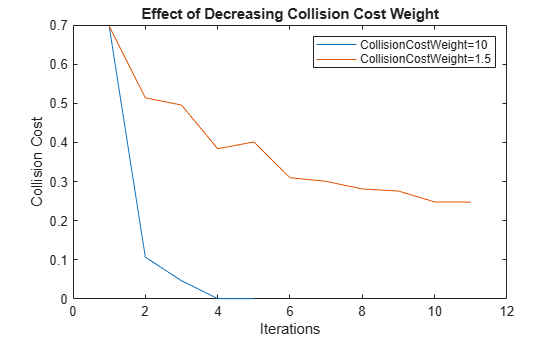#### Increase Smoothness Cost Weight

Increase the smoothness cost weight to a value greater than the collision cost weight, indicating that smoothness is of a higher priority. Then, plan a place trajectory.

```chomp.SmoothnessOptions.SmoothnessCostWeight = 0.1; chomp.CollisionOptions.CollisionCostWeight = 1e-3; [placetraj,~,highsmoothinfo] = optimize(chomp,[pickConfig; placeConfig], ... [0 2], ... 0.10, ... InitialTrajectoryFitType="minjerkpolytraj");```

Plot the results for the smoothness cost weights of `1e-3` and `10` to see the effect. Note that setting the smoothness cost weight higher results in a higher collision cost when the optimizer completes its trajectory planning. When smoothness cost weight is lower, notice that the smoothness cost increases because the manipulator must take a longer path in the workspace to prioritize collision avoidance.

```figure plot([solplaceinfo.InitialSmoothnessCost,solplaceinfo.SmoothnessCost]) hold on plot([highsmoothinfo.InitialSmoothnessCost,highsmoothinfo.SmoothnessCost]) title("Effect of Decreasing Smoothness Cost Weight") legend({"SmoothnessCostWeight=1e-3","SmoothnessCostWeight=10"}) xlabel("Iterations") ylabel("Smoothness Cost") hold off```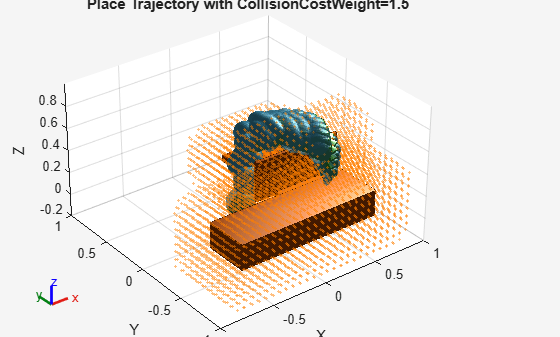Display the `IsCollisionFree` field of the solution info and show the trajectory with the higher smoothness cost weight. Notice that the trajectory is no longer collision free after increasing the smoothness cost weight to `10`.

`disp(highsmoothinfo.IsCollisionFree)`
``` 0 ```
```show(chomp,placetraj); title("Place Trajectory with SmoothnessCostWeight=1.5") xlim([-1 1]) ylim([-1 1]) zlim([-0.2 1])```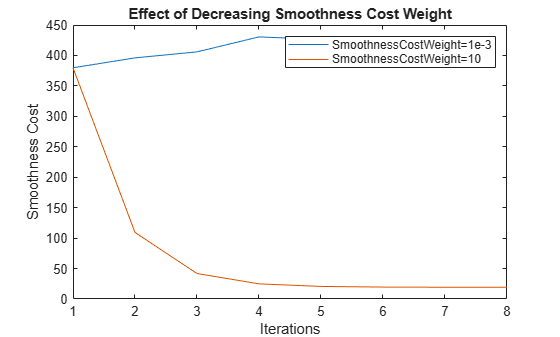#### Decrease Learning Rate

Decreasing the learning rate, while keeping the original smoothness and collision cost weights, results in the solver requiring more iterations for the solution to converge. Decrease the learning rate and return the collision and smoothness costs to their original values. Then, plan a place trajectory using the solver, and visualize the required iterations for the solver with the original learning rate and the decreased learning rate.

```chomp.CollisionOptions.CollisionCostWeight = 10; chomp.SmoothnessOptions.SmoothnessCostWeight = 1e-3; chomp.SolverOptions.LearningRate = 0.5; [placetraj,~,lowlearningrateinfo] = optimize(chomp,[pickConfig; placeConfig], ... [0 2], ... 0.10, ... InitialTrajectoryFitType="minjerkpolytraj"); figure plot([lowlearningrateinfo.InitialObjectiveFunction,lowlearningrateinfo.ObjectiveFunction]) hold on plot([solplaceinfo.InitialObjectiveFunction,solplaceinfo.ObjectiveFunction]) title("Effect of Decreasing Learning Rate") legend({"LearningRate=0.1","LearningRate=5"}) xlabel("Objective Function") ylabel("Iterations")```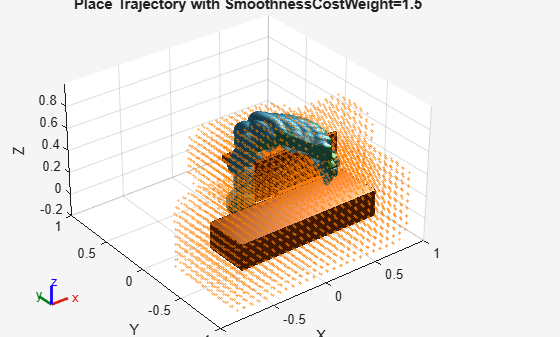### Visualize Trajectory

Visualize the picking and placing trajectories of the robot.

```figure subplot(1,2,1) show(chomp,optimpickconfig,CollisionObjects=env); xlim([-1 1]) ylim([-1 1]) zlim([-0.2 1]) view([pi 0 0]) title("Picking Trajectory") subplot(1,2,2) show(chomp,optimplaceconfig,CollisionObjects=env); xlim([-1 1]) ylim([-1 1]) zlim([-0.2 1]) title("Placing Trajectory") view([pi 0 0])```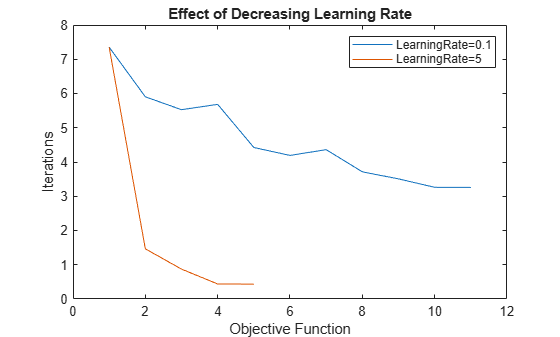### Visualize Robot Motion

Animate the trajectory of the robot for the entire pick-and-place task.

```figure show(franka,startConfig,Collisions="on",Visuals="off"); title("Animated Pick-and-Place Trajectory") xlim([-1 1]) ylim([-1 1]) zlim([-0.2 1]) rc = rateControl(5); view([pi 0 0]) hold on for i = 1:length(env) if i == indexOfCollisionObjectInEnvironmentToPick [~,p] = show(env{i}); % Store patch of initial soda can location else show(env{i}); end end exampleHelperVisualizePickTrajectory(franka,optimpickconfig,rc); exampleHelperVisualizePlaceTrajectory(franka,env{indexOfCollisionObjectInEnvironmentToPick},optimplaceconfig,rc,p); hold off```